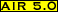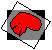### AIR Internal Coordinate System

The internal coordinate system used by the AIR package will not necessarily assign the same axes or voxel coordinates that your image display package assigns. The internal coordinate axes of an image file are defined by the order in which the image voxels are stored in the file with the x-dimension changing most often (with every voxel) and the z-dimension least often. When an image file is loaded, the center of the first voxel in the file is assigned the internal (x,y,z) coordinates (0,0,0). Assuming that the x-dimension of the image matrix is greater than 1, the center of the second voxel in the file is assigned the internal coordinates (1,0,0). After a number of pixels equal to the file x-dimension (as specified in the corresponding header file) has been loaded, the next voxel is assigned the internal coordinates (0,1,0),etc. The center of the last voxel in the file is assigned coordinates (x-dimension -1,y-dimension -1,z-dimension -1). The legal range for each coordinate is as follows:

• x-coordinate: 0 to x-dimension -1
• y-coordinate: 0 to y-dimension -1
• z-coordinate: 0 to z-dimension -1

The internal coordinate system is the one referenced by the homogenous transformation matrix contained in the .air files.

Your image display package may assign different coordinates to voxels for a number of reasons:UCF files do make explicit reference to the AIR coordinate system. In these files, coordinates must be converted from units of voxels to real world units. Given an image file's real world voxel dimensions of x_size, y_size and z_size, the AIR internal coordinate in voxel units (x, y, z) can be converted by simple multiplication to the AIR internal coordinate in real world units (x*x_size, y*y_size, z*z_size).Modified: December 15, 2001